# Negative Feedback in Op amp and Closed Loop Gain of Op amp

## Negative Feedback in Op Amp

We obtain Negative feedback in an op amp by connecting output terminal of an op amp to its inverting input terminal through a suitable resistance as shown below.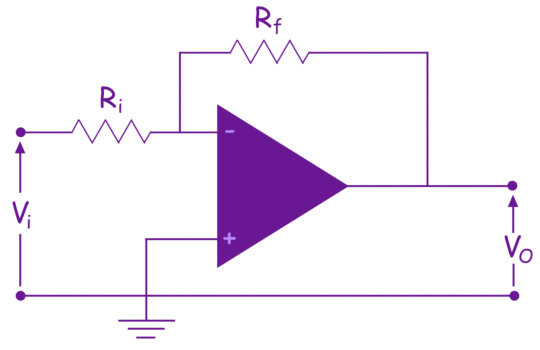The gain of an op amp with negative feedback is called closed loop gain.

## Closed Loop Gain of Op Amp

When we connect a feedback resistance and a resistance in series with the inverting input terminal of an op-amp as shown in the above picture, the gain of the system just becomes the negative ratio of feedback resistance to input resistance. The operational amplifier has its own gain. The gain is practically very large and ideally, it is infinity. We can impose a predetermined gain to the system irrespective of op amp’s own gain (open loop gain). We do this by choosing the appropriate values of series input resistance (Ri) and feedback resistance (Rf). The gain of the op-amp system should be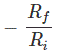To understand the closed loop gain of an 741 op amp, let us go through an example. 741 operational amplifier has following perameters.

 Parameter Value Open Loop Gain 2 × 105 Input Resistance 2 MΩ Output Resistance 5 Ω

Let us find the closed loop gain of the op amp when we connect a 10 kΩ resistance in series with the inverting terminal and a 20kΩ resistance as feedback path.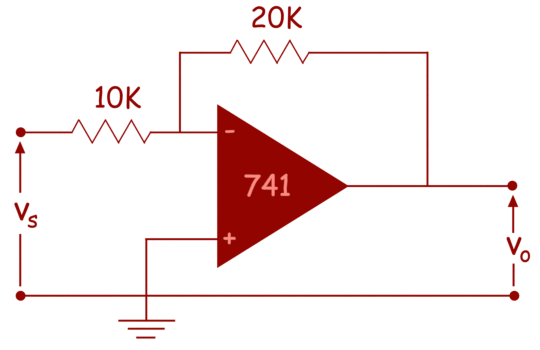The equivalent circuit of the op amp with input source will be as shown below,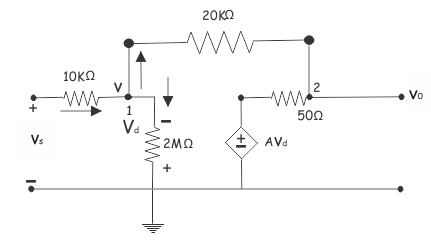Let us assume, the voltage at node 1 is v. Now applying Kirchhoff current law at this node. we get,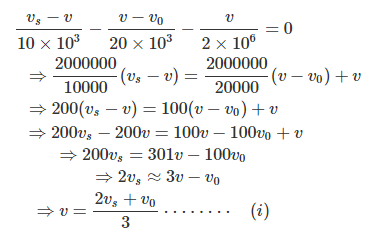Now by applying Kirchhoff current law at node 2 get,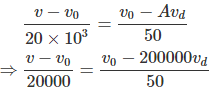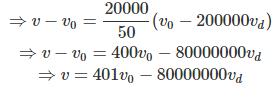Now, from the figure it is found that,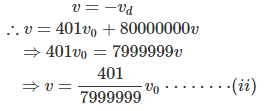From equation (i) and (ii) we get,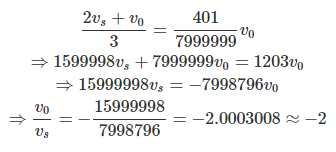So, the open loop gain of the op amp is, 2 × 105.
whereas closed loop gain comes to only 2.

Let us take another example of closed loop gain of an op amp.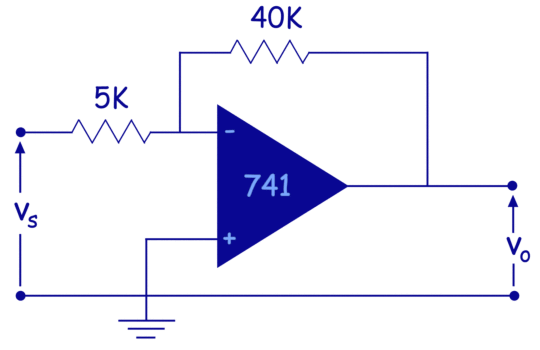The equivalent circuit of above 741 op amp circuit can be redrawn as,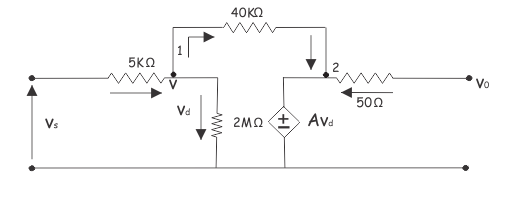Now, consider voltage at node 1 is v applying Kirchhoffs current law at node 1. We get,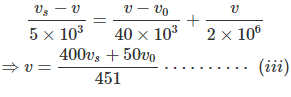Now, applying Kirchhoffs current law at node of the equivalent circuit, We get,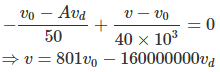Now,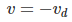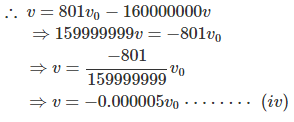From the equation (iii) and (iv), we get,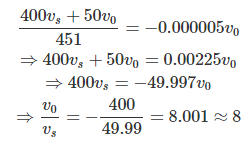So, the closed loop gain of the above op amp circuit is 8.
So, it is cleared that by choosing suitable resistor for feedback in an op amp circuit, we can impose its desired gain.

Want To Learn Faster? 🎓
Get electrical articles delivered to your inbox every week.
No credit card required—it’s 100% free.